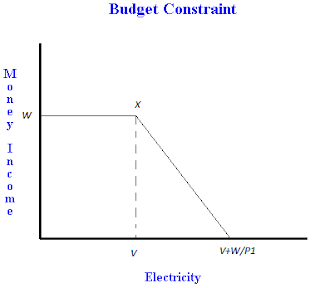Budget constraint with an endowment (a free amount of one good) - FreeEconHelp.com, Learning Economics... Solved!

## 11/16/11

This economics entry goes over a budget constraint example where the individual in question receives a free amount of the good to begin with.  The way these types of problems are modeled is slightly different from our typical budget constraint problem.  Another common application for this type of problem is the introduction of food stamps or low income housing.  Any microeconomics problem where the individual is given a free amount of the good will have a similar solution to the one shown below.  See the graphs and explanations below for more information, and here is the question:

An Employee of American Electricity PLC receives income partly in form of money salary of \$W per month and partly in form of free electricity allowance (a fixed number of Kilowatts a month).  If she wants, she can buy more electricity at the normal price P1 per kilowatts but for technical reasons she cannot resell the electricity. The value of her free electricity at the normal price is \$V so her total remuneration package is worth \$X= \$(V+W).

1.Draw a budget constraint to reflect this monthly Salary package with money income on the vertical axis and electricity on the horizontal axis clearly identifying \$X, \$V and \$ W.

2. On a separate diagram, reproduce the budget constraint and draw a selection of indifference curves that illustrate the situation where an increase in the price of electricity (from P1 to P2) causes the employee to reduce her electricity consumption.

3. Reproduce the first diagram and draw an indifference curve to illustrate the case in which the employee would be indifferent to her current remuneration package and a straight monetary salary of \$Y where \$Y < \$X.  You may assume here that the price of electricity is back at the normal level of P1.The trick to answering number 1 is two recognize that a certain amount of the good is given freely.  We show this in the budget constraint with a straight line.  This means that the individual can have up to \$V of electricity (for free) but they do not have the ability to substitute it for money income.  You can see that the maximum for money income is \$W, while the maximum for electricity is \$V + \$W/P1.  This shows that money income can be used to purchase electricity, but it cannot go the other way.  The variable \$X is shown off of the axis, because it is the combination of \$W and \$V, and only occurs when \$W is used for money (exclusively).  If any amount of \$W is used to buy electricity, we are no longer at \$X.

For the second question we need to increase the price of electricity.  We can do this by rotating the budget constraint in.  Remember that the free electricity is the same amount (\$V) but the act of substituting money income for electricity has become more expensive.  This results in less electricity being able to be bought at the maximum, and if the indifference curves are well behaved we will see less electricity being consumed and more money income being held.

Finally, we need to show a situation where the individual is indifferent between their current compensation package, and one where the total salary offered is less than \$X, but does not include free electricity.  This means that the person is freely allowed to allocate their budget, rather than being forced (because it is free) to consume a certain amount of electricity.  The person will only be indifferent to the two if the highest indifference curve touches the pivot point of the budget constraint.  When this occurs, it tells us that the person in question really likes to hold money income and is not willing to trade any of it for electricity.  In fact, the person would like to trade electricity for money, but since that is not allowed, they settle for the pivot point.  You can see from the graph that the same indifference curve is tangent to the pivot point of the first budget constraint line, and is also tangent to the second budget constraint line even though the second budget constraint line clearly shows a lower income level.  This result shows the power of choice in determining optimum utility (choice can be more powerful than money :)  ).

Remember, this type of problem can also apply to things like food stamps, free housing, or other government program (possibly trust funds) where the choosing power of the agent in question is limited.